International
Tables for
Crystallography
Volume B
Reciprocal space
Edited by U. Shmueli

International Tables for Crystallography (2006). Vol. B. ch. 4.5, pp. 468-469   | 1 | 2 |

## Section 4.5.2.3.2. Diffraction by helical structures

R. P. Millanea*

#### 4.5.2.3.2. Diffraction by helical structures

| top | pdf |

Denote by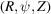a cylindrical polar coordinate system in reciprocal space (with the Z and z axes parallel), and by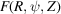the Fourier transform of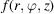. Sinceis periodic in z with period c, its Fourier transform is nonzero only on the layer planes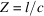where l is an integer. Denote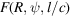by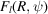; using the cylindrical form of the Fourier transform shows that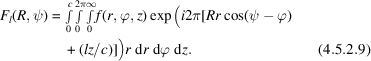It is convenient to rewrite equation (4.5.2.9)making use of the Fourier decomposition described in Section 4.5.2.3.1, since this allows utilization of the helix selection rule. The Fourier–Bessel structure factors (Klug et al., 1958),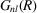, are defined as the Hankel transform of the Fourier coefficients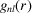, i.e.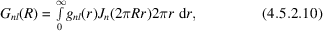and the inverse transform is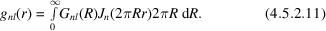Using equations (4.5.2.7)and (4.5.2.11)shows that equation (4.5.2.9)can be written as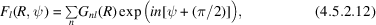where, as usual, the sum is over only those values of n that satisfy the helix selection rule. Using equations (4.5.2.8)and (4.5.2.10)shows that the Fourier–Bessel structure factors may be written in terms of the atomic coordinates as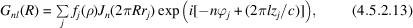where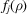is the (spherically symmetric) atomic scattering factor (usually including an isotropic temperature factor) of the jth atom and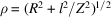is the spherical radius in reciprocal space. Equations (4.5.2.12)and (4.5.2.13)allow the complex diffracted amplitudes for a helical molecule to be calculated from the atomic coordinates, and are analogous to expressions for the structure factors in conventional crystallography.

The significance of the selection rule is now more apparent. On a particular layer plane l, not all Fourier–Bessel structure factorscontribute; only those whose Bessel order n satisfies the selection rule for that value of l contribute. Since any molecule has a maximum radius, denoted here by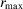, and since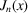is small for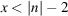and diffraction data are measured out to only a finite value of R, reference to equation (4.5.2.10)[or equation (4.5.2.13)] shows that there is a maximum Bessel order that contributes significant value to equation (4.5.2.12)(Crowther et al., 1970; Makowski, 1982), so that the infinite sum over n in equation (4.5.2.12)can be replaced by a finite sum. On each layer plane there is also a minimum value of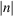, denoted by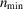, that satisfies the helix selection rule, so that the region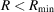is devoid of diffracted amplitude where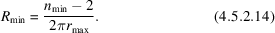The selection rule therefore results in a region around the Z axis of reciprocal space that is devoid of diffraction, the shape of the region depending on the helix symmetry.

### ReferencesCrowther, R. A., DeRosier, D. J. & Klug, A. (1970). The reconstruction of a three-dimensional structure from projections and its application to electron microscopy. Proc. R. Soc. London Ser. A, 317, 319–344.Google ScholarKlug, A., Crick, F. H. C. & Wyckoff, H. W. (1958). Diffraction from helical structures. Acta Cryst. 11, 199–213.Google ScholarMakowski, L. (1982). The use of continuous diffraction data as a phase constraint. II. Application to fibre diffraction data. J. Appl. Cryst. 15, 546–557.Google Scholar